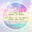# Chapter F1TAI SI EN AMELIA .
Get Started. It's FreeChapter F1## 1. Force

### 1.1. Definition

1.1.1. Force is a push or a pull.

### 1.2. Effects

1.2.1. Change direction of motion of an object

1.2.2. Cause a (stationary) object to move/stop

1.2.3. Change an object's physical form

1.2.4. Frictional force

1.2.4.1. Able to walk without slipping

1.2.5. Gravitational force

1.2.5.1. Allow us to remain on the ground and not float in mid-air

### 1.3. Types of forces

1.3.1. Frictional force

1.3.2. Gravitational force

1.3.3. Elastic spring force

1.3.4. Magnetic force

1.3.5. Air resistance

### 1.4. Measuring force

1.4.1. Use electronic balance to determine the object's mass, use accelerometer to measure the object's acceleration. Formula=Mass×Acceleration

### 1.5. Importance

1.5.1. Frictional force(Friction)

1.5.1.1. Slows down/stops a moving object. You won't want to continue chasing after your run-away tennis ball right?

1.5.1.2. Produces heat

1.5.2. Gravitational Force(Gravity)

1.5.2.1. Pulls an object down so it will not float in the air

1.5.3. Magnetic force

1.5.3.1. Can reduce friction and cause objects to move faster when needed. (E.g a type of train called the maglev train)

### 1.6. SI unit

1.6.1. Newton (N)

## 2. Pressure

### 2.1. Definition

2.1.1. The force per unit area exerted on an object

### 2.2. How to increase pressure

2.2.1. There's more force applied on an object

2.2.2. For the same force, pressure is higher when a smaller surface is in contact with an object.

### 2.3. How to decrease pressure

2.3.1. By reducing the amount of force applied on an object

2.3.2. Pressure exerted is lower when a larger surface is in contact with the ground or an object.

### 2.4. Calculating pressure

2.4.1. Formula=Force÷Area

### 2.5. SI unit

2.5.1. Pascal (Pa)